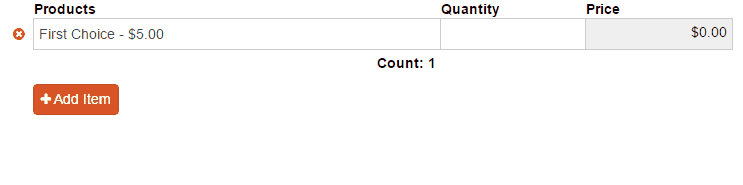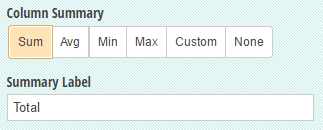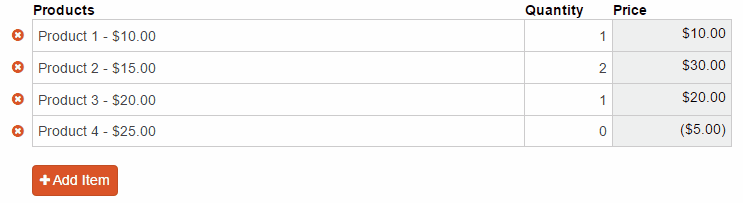# Table Field Tips & TricksBy Nadia A. | October 17, 2016

Table fields are an awesome way to collect a large amount of repeating data in a compact space. In a previous post, we walked through the process of creating a fairly basic expense report using a table field. In this post, we’re going to explore some of the more complex uses of a table field:

You can almost think of table fields and repeating sections as twins - but they’re not identical. Other than the concise grid format, what really sets table fields apart from repeating sections is the ability to add a summary and label at the bottom of each column. These summaries can be basic, like a running count of total items added:Or, you can create a custom summary using a calculation; like adding up the number of times a specific choice option is selected:

`=Table.Count(Choice="Second Choice")`

Plus, for columns that collect payment, you can use the column summary to perform a variety of functions without having to write any calculations - such as summing a price total:## Referencing table field data

Typically, when you want to reference data from another field, you can just use a simple default value:

`=Textbox`

Or, if the field is in a section:

`=Section.Textbox`

However, targeting column data from outside of a table field is a little bit different. Because a table field contains potentially unlimited rows of repeating data, your calculation will need to be more specific. For example, if one of the columns in your table is an Email field, you can use the .Select function to compile a list of emails from every row in that column:

`=Table.Select(Email))`

But what if you want to target an email from just one row? To to this, you’ll need to reference that row’s item number:

`=Table.Where(ItemNumber = 1).Select(Email))`

Now, only the inputted email from the very first row will appear. If you were to add a second Email field outside of your table and use this expression as the default value, you could set then set this email as To address in your email confirmations.

## Creating conditional values

Let’s say your business is using a table field to sell an assortment of products. As an incentive for your customers, you may want to offer a reduced price depending on the number of different products that a customer orders. After you set your table up with a Products column and a Quantity column, try adding a Price column with the following expression set as the Amount:

`=if ItemNumber > 3 then (Quantity*Products_Amount-5) else Quantity*Products_Amount`

So, what exactly is going on here?

The beginning of our if/then statement references the Item Number - in this case, the discount will apply once the customer adds more than 3 items (at least 4) to their order. If they do have 4 or more items, then the total price will be reduced by \$5.00 for every row starting with the fourth item:There are many other useful functions you can accomplish with the help of if/then statements. For example, you can discount items by quantity rather than the total number of orders:

`= if Quantity > 3 then (Products_Amount*Quantity)-5 else Products_Amount*Quantity`

Here, if the customer orders at least 4 of the same item, they’ll receive a \$5 discount on that item. If they order less than 4, the item will be regularly priced. You can even get more specific and give a different discount depending on which item is selected:

`= if Form.Order1.Select(Products).Contains("Product 1") and Quantity > 3 then 5*Quantity else Products_Amount*Quantity`

This expression essentially states: if the customer selects at least 4 of Product 1, the price will become \$5 multiplied by the item quantity. If they purchase less than 4, the price will just be the regular assigned price multiplied by the item quantity. You can, of course, adjust these numbers as needed on your own form.